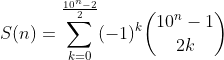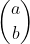# Problem 52729. Easy Sequences 21: Combinatorial Summations

Create the function S(n), defined by the following summation:The symbolis the combination function ('nchoosek(a,b)' in MATLAB). 'S(n)' always yields an integer value, if 'n' is a positive integer. Present the output modulo 1234567.
NOTE: S(n) may have positive or negative values. Therefore, please use the 'mod' function instead of the 'rem' function to ensure the result would be a positive modulus.

### Solution Stats

69.23% Correct | 30.77% Incorrect
Last Solution submitted on Jul 17, 2023

### Community Treasure Hunt

Find the treasures in MATLAB Central and discover how the community can help you!

Start Hunting!